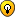## James Bond Killer

For fans of Killer Sudoku, Samurai Sudoku and other variants

### James Bond Killer

Based on an idea of Richard.
Fill in the grid with numbers 0,0,1..7
It should be a real insane, but no long T&E needed to solve it.Have funJean-Christophe

Posts: 149
Joined: 22 January 2006

And for people who like the character, here is a themed-version:Click on the little pic to view the large pic...(The file size of the large pic is about 180kB...)
udosuk

Posts: 2698
Joined: 17 July 2005

I must admit, I first forgot to consider a case and it came out harder to solve then I wanted.

Anyhow, it's still solvable by logic. Here is my walktrhough :

Step 1
Cage 17/3 in N4 = {467}
Innies of N5 -> R4C6+R6C4 = 12 = {57}, naked pair in D/, N5
In&Outies of C12 -> R9C34-R78C2 = 13
Max R9C34 = {67} = 13 -> Max R78C2 = 13-13 = 0 = Min R78C2
-> R78C2 = , R9C34 = {67}, naked pair in R9 and also in cage 28/7
-> {67} of N7 locked in R789C3 -> not elsewhere in C3
Cage 4/3 in N8 <> {004} = {013}
-> no {13} elsewhere in R8, N8, no 0 elsewhere in R8, only one 0 elsewhere in N8

Step 2
Cage 28/7 in N7 = {1234567}, must include a 1
Cage 18/7 in N7 = {0012357|0012456}, must include a 1 in R67C3
Both cages must include a 1 -> one of the 1 must go out of N7 in R6C13
-> no 1 elsewhere in R6, N4

Step 3
Outies of N7 -> R6C134+R79C4 = 18
Min R69C4 = {56} = 11 -> Max R6C13+R7C4 = 18-11 = 7
Max R69C4 = {67} = 13 -> Min R6C13+R7C4 = 18-13 = 5
-> R6C13+R7C4 = 5..7
-> R6C13 = {12|13} = 3|4, R7C4 = {24}

Step 4
Cage 28/7 in N7 = {1234567} with {45} locked in N7
-> R78C3 <> {45}
Cage 18/7 in N7 = {0012357|0012456} = {00125...}, must include a 5
-> R6C4 = 5, R4C6 = 7
-> R79C4 = 9|10 = {27|46}

Step 5
1 of Cage 18/7 locked in R67C3 -> not elsewhere in C3
{67} of N7 locked in R789C3 -> not elsewhere in C3
4 of C3 locked in R123C3 -> not elsewhere in N1

Step 6
Innies of N124 -> R13C6+R6C13 = 6
-> R13C6 = 2|3 = {02|03|12}
Cage 24/7 in N3 = {0024567|0123567} = {2567...}
Cage 23/7 in N3 = {0014567|0023567|0123467} = {67...}
Both cages must include a 6 -> one of the 6 must go out of N3 in R3C79
-> no 6 elsewhere in R3, N6
6 of C8 locked in R123C8 -> not elsewhere in N3

Step 7
Outies of N3 -> R13C6+R4C79 = 12
-> R4C79 = 9|10 = {36|46}
5 of Cage 24/7 locked in N3 -> not elsewhere in N3
-> Cage 23/7 = {0123467}
Both cages must include a 2 -> one of the 2 must go out of N3 in R13C6 = {02|12}

Step 8
Cage 12/4 in N14 = {0057|0156|0237|1236} = {(6|7)...}
R4C2 = {235} <> {0716} -> R234C1 <> 5

Step 9
In&Outies of R1234 -> R4C458-R5C3 = 4 = {0013|0125|1223|1234|1355} = {(2|3)...}
-> R4C12 <> {23} = [02|03|05] -> R4C1 = 0

Step 10
R345C3 <>  = {002|003|023|024|025|235|245} = {(2|3)...}
R678C3 = {126|137} = {(2|3)...}
R345C3 & R678C3 forms a complex naked pair on {23}
-> R12C3 <> {23} = {05|45} = {5...} -> no 5 elsewhere in C3, N1, R1C45
-> R4C2 = 5
Cage 12/4 in N1 = {0237|1236} = {23...} -> no {23} elsewhere in N1

Step 11
Cage 18/4 in N12 = {0567|3456} -> R1C45 = {36|67} = {6...}
-> 6 of Cage 18/4 locked in R1C45 -> not elsewhere in N2, R1
-> R4C9 = 6 (hidden single in Cage 24/7)
6 of N9 locked in R78C7

Step 12
Outies of C89 -> R123C7 = 8 = {017|035|125|134}
R4C7 = {34} -> R123C7 <> {134}, R1C7 = {57}

Step 13
Cage 6/4 in N9 = {0015|0024|0123}
R8C8 = {245} <> {001} -> R7C8,R9C89 <> 5
-> 5 of R9 locked in Cage 13/4 in N89 = {0157|0256} = {0(6|7)...}
-> R8C7 = {67}
4 of N8 locked in R7C456 -> not elsewhere in R7
4 of C7 locked in R456C7 -> not elsewhere in N6
5 of D\ locked in R7C7&R8C8 -> not elsewhere in N9
5 of R9 locked in R9C56 -> not elsewhere in N8

Step 14
Cage 14/4 in N69 = {0347|1247} = {47...}
-> R8C9 = 4
Cage 6/4 in N9 = {0015|0123} = {01...}
R9C567 = {025} -> R9C3 = 6

Step 15
R89C3 = , R79C4 = 
R9C7 = 2, R8C17 = 
R9C56 = {05}, R9C12 = {34}, R67C1 = 
R67C3 = 
Cage 14/4 in N69 = {0347}, R7C9 = 3, R7C7 = 7
R7C56 = {46}, R56C7 = , R6C89 = 
Cage 10/3 in N6 = {235}, R5C9 = 5, R4C7 = 4
...
Jean-Christophe

Posts: 149
Joined: 22 January 2006